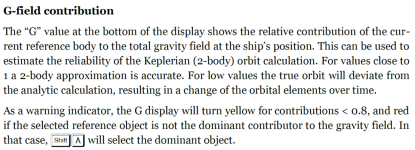# General QuestionTrue and analytic orbit in Orbiter

#### misha.physics

##### Member
Hi,

I'm confused a lot. Wikipedia says that Orbier uses n-body Newtonian mechanics for simulation. But then how to understand written in the Orbiter manual this?:Or does it not mean that Orbiter uses a 2-body approximation during simulation? For example, what parameters does show Orbit MFD, namely true (with considering n-body gravitation interction in the Solar System) or approximate (2-body)?

#### Urwumpe

##### Not funny anymore
Donator
It uses "Osculating elements" for its Orbit MFD navigation display.

That are the kepler elements, which represent the current state vector and approximates its future trajectory. Thus they change all the time and are recomputed from the newtonian solution.

•misha.physics

#### Sbb1413

Hi,

I'm confused a lot. Wikipedia says that Orbier uses n-body Newtonian mechanics for simulation. But then how to understand written in the Orbiter manual this?:

View attachment 27722

Or does it not mean that Orbiter uses a 2-body approximation during simulation? For example, what parameters does show Orbit MFD, namely true (with considering n-body gravitation interction in the Solar System) or approximate (2-body)?
Although I might understand this, I can't explain this well. And I won't do this for now as I have been busy in recent months.

•misha.physics

#### misha.physics

##### Member
It uses "Osculating elements" for its Orbit MFD navigation display.

That are the kepler elements, which represent the current state vector and approximates its future trajectory. Thus they change all the time and are recomputed from the newtonian solution.
Thank you. It seems I undestand now. Orbital flight in Orbiter is running in accordance to a solution of a n-body newtonian dynamic system. But since we can't have an analytical solution for n>2 body gravitation system, we can't draw the same orbit for all times. Thus, we plot an osculating orbit "step by step" using the current state vectors (position and velocity) and the resulting gravitation force (acceleration) which includes the influence of all gravitation bodies (Sun, Earth, Moon, Saturn, Io and so on). And the orbit and its parameters given in Orbit MFD change all the time. So the movement itself in Orbiter occurs in accordance to a n-body dynamic system, but an orbit image and its parameters are recalculated for every time using an analytical solution for 2 bodies (Kepler's equation for the two-body problem) and we see it, for example, in Orbit MFD.

Does I understand correctly?

•N_Molson and Sbb1413

#### Urwumpe

##### Not funny anymore
Donator
Yes, thats it.

•misha.physics